### GATE 2013 ECE Video Solutions on Analog Circuits (Analog Electronics)

1. In the circuit shown below, what is the output voltage (Vout), if a silicon transistor Q and an ideal OP-AMP are used?a. - 15 volts
b. - 0.7 volts
c. + 0.7 volts
d. + 15 volts
Solution: https://www.youtube.com/watch?v=vR9F9Cmapog

2. In a voltage – voltage feedback as shown below, which one of the following statements is TRUE, if the gain K is increased?a. The input impedance increases and output impedance decreases
b. The input impedance increases and output impedance increases
c. The input impedance decreases and output impedance decreases
d. The input impedance decreases and output impedance increases
Solution: https://www.youtube.com/watch?v=3SI-KyaLnm8

3. The small signal resistance (ie. dVB/dID) in KΩ offered by the n – channel MOSFET M shown in the figure below, at a bias point of VB = 2 volts is (device data for M: device Transconductance parameter KN = µNCOX(W/L) = 40 µA/V2, threshold voltage VTN = 1 volt, and neglecting body effect and channel length modulation effects)a. 12.5
b. 25
c. 50
d. 100
Solution: https://www.youtube.com/watch?v=78GP87ThDTg

4. The ac schematic of an NMOS common source stage is shown in the figure below, where part of the biasing circuit has been omitted for simplicity. For the n – channel MOSFET M, the Transconductance gm = 1 mA/V and body effect and channel length modulation effect are to be neglected. The lower cutoff frequency in Hz of the circuit approximately ata. 8
b. 32
c. 50
d. 200
Solution: https://www.youtube.com/watch?v=2-LoL607LVE

5. In the circuit shown below, the OP-AMPs are ideal. Then Vout in volts isa. 4
b. 6
c. 8
d. 10
Solution: https://www.youtube.com/watch?v=txQhRQhmZfE

6. A voltage 1000sinωt volts is applied across YZ. Assuming ideal diodes, the voltage measured across WX in volts is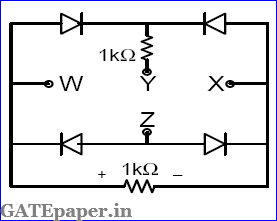a. Sinωt
b. (Sinωt + |Sinωt|) / 2
c. (Sinωt - |Sinωt|) / 2
d. 0 for all t
Solution: https://www.youtube.com/watch?v=gy9k16uKPDk

7. In the circuit shown below, the knee current of ideal zener diode is 10 mA. To maintain 5 volts across the load RL, the minimum value of RL in Ω’s and the minimum power rating of the zener diode in mW respectively area. 125 and 125
b. 125 and 250
c. 250 and 125
d. 250 and 250
Solution: https://www.youtube.com/watch?v=_QUM3VQQ78I

8. In the circuit shown below, the silicon NPN transistor Q has a very high value of β. The required value of R2 in KΩ to produce IC = 1 mA is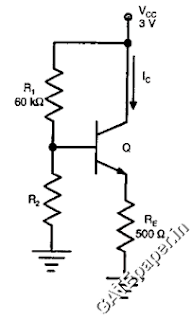a. 20
b. 30
c. 40
d. 50
Solution: https://www.youtube.com/watch?v=seLmVm94TqY

### GATE 1991 ECE Video Solutions on Digital Circuits (Digital Electronics)

1. Two dimensional addressing of 256 X 8 bit ROM using 8 to 1 selectors requires _______ (how many?) NAND gates.
Solution: https://www.youtube.com/watch?v=6TdeOmgMTvs

2. The CMOS equivalent of the following nMOS gate (shown in figure) is ________ (Draw the circuit).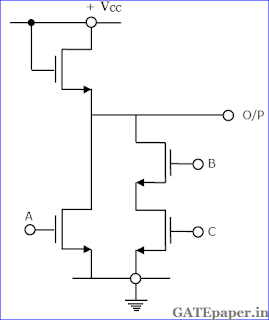Solution: https://www.youtube.com/watch?v=9cSuZt-UrPo

3. A bit stored in a FAMOS device can be erased by ___________.
Solution: https://www.youtube.com/watch?v=mwBl4KpMcLs

4. In the figure, the Boolean expression for the output in terms of inputs A, B and C when the clock CK is high, is given by ____________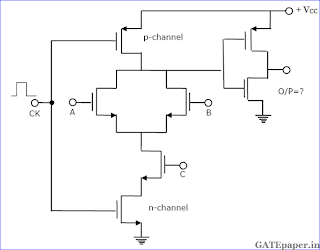Solution: https://www.youtube.com/watch?v=cbPlHJk9zNA

5. The program given below is run on an 8085 based microcomputer system. Determine the contents of the registers: PC, SP, B, C, H, L after a halt instruction is executed.Answer:
Solution:

6. The four variable function f is given in terms of min-terms as f(A, B, C, D) = ∑m(2,3,8,10,11,12,14,15). Using the K-map minimize the function in the sum of products form. Also, give the realization using only two input NAND gates.
Solution: https://www.youtube.com/watch?v=x_pBfG9g-8w

7. An S-R flip flop can be converted into a T flip flop by connecting ____ to Qbar and _____ to Q.
Solution: https://www.youtube.com/watch?v=sxSeKlxdxSc

### GATE 1992 ECE Video Solutions on Digital Circuits (Digital Electronics)

1. The logic realized by the circuit shown in figure isSolution: https://www.youtube.com/watch?v=ZDCopbfFlqo

2. Choose the correct statement(s) from the following:
a. PROM contains a programmable AND array and a fixed OR array
b. PLA contains a fixed AND array and a programmable OR array
c. PROM contains a fixed AND array and a programmable OR array
d. PLA contains a programmable AND array and a programmable OR array
Solution: https://www.youtube.com/watch?v=z2tByyE5L24

3. The figure shows the circuit of a gate in the Resistor Transistor Logic (RTL) family.The circuit represents a
a. NAND
b. AND
c. NOR
d. OR
Solution: https://www.youtube.com/watch?v=7EU2sl8UvK8

4. The initial contents of the 4 bit serial in serial out, right shift, shift register shown in figure, are 0110. After three clock pulses are applied, the contents of the shift register will be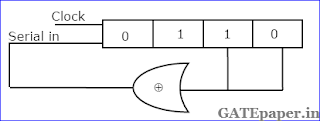a. 0000
b. 0101
c. 1010
d. 1111
Solution: https://www.youtube.com/watch?v=0VtDZLpnhOM

5. In an 8085 microprocessor system with memory mapped I/O,
a. I/O devices have 16 bit addresses
b. I/O devices are accessed using IN and OUT instructions
c. There can be a maximum of 256 input devices and 256 output devices
d. Arithmetic and logic operations can be directly performed with the I/O data.
Solution:

6. The following program is run on 8085 microprocessor:At the completion of the execution of the program, the program counter of the 8085 contains _____________ and the stack pointer contains _______________.
Answer:
Solution:

7. A new clocked X-Y flip flop is defined with two inputs, X and Y is in addition to the clock input. The flip flop functions as follows:
If XY = 00, the flip flop changes state with each clock pulse
If XY = 01, the flip flop state Q becomes ‘1’ with the next clock pulse
If XY = 10, the flip flop state Q becomes ‘0’ with the next clock pulse
If XY = 11, the change of state occurs with the clock pulse
a. Write the truth table for the XY flip flop
b. Write the excitation table for the XY flip flop
c. It is desirable to convert a J-K flip flop into X-Y flip flop by adding some external gates, if necessary. Draw a circuit to show how you will implement in X-Y flip flop using a J-K flip flop.

Solution: https://www.youtube.com/watch?v=c8PZBGHCGDQ

8. Dual slope integration type analog to digital converter provide
a. Higher speeds compared to all other types of A/D converters
b. Very good accuracy without putting extreme requirements on component stability
c. Good rejection of power supply hum
d. Better resolution compared to all other types of A/D converters for the same number of bits.
Solution: https://www.youtube.com/watch?v=aN33UvCFYhs

9. A combinational circuit has three inputs A, B and C and an output F. F is true only for the following combinations.
A is false and B is true
A is false and C is true
A, B and C are all false
A, B and C are all true
a. Write the truth table for F. Use convention, true = 1 and false = 0.
b. Write the simplified expression for F as a sum of products.
c. Write the simplified expression for F as a product of sums.
d. Draw the logic circuit implementation of F using the minimum number of 2 input NAND gates only.

Solution: https://www.youtube.com/watch?v=woAddE4waaU

10. Figure shows the memory circuit of 8085 microprocessor.a. What is the total size of the memory in the circuit?
b. What are the beginning and ending addresses of the memory in chip 1?
c. What are the beginning and ending addresses of the memory in chip 2?
d. Are the memory chips in the circuit ROM or RAM?
e. How will you replace the two NAND gates I the circuit with one 3 to 8 decoder without changing the memory size or the memory addresses? Assume that the decoder has one active high enable E1 and one active low enable E2.

Solution: https://www.youtube.com/watch?v=ZhLawfMasrY

### GATE 1993 ECE Video Solutions on Digital Circuits (Digital Electronics)

1. For the logic circuit shown in figure, the output Y is equal toSolution: https://www.youtube.com/watch?v=yv328aRJc8o

2. The truth table for the output Y in terms of three inputs A, B and C are given. Draw a logic circuit realization using only NOR gates.Solution: https://www.youtube.com/watch?v=TVdLzstLObk

3. 2’s complement representation of a 16 bit number (one sign bit and 15 magnitude bits) is FFFF. Its magnitude in decimal representation is
a. 0
b. 1
c. 32,767
d. 65,535
Solution: https://www.youtube.com/watch?v=4ZrEe07cDuQ

4. Boolean expression for the output of XNOR logic gate with inputs A and B is
a. AB’ + A’B
b. (AB)’ + AB
c. (A’ + B)(A + B’)
d. (A’ + B’)(A + B)
Solution: https://www.youtube.com/watch?v=m0IvVDDvYtE

5. A pulse train with a frequency of 1 MHz is counted using a modulo 1024 ripple counter built with JK flip-flops. For proper operation of the counter, the maximum permissible propagation delay per flip flop stage is ____ nsec.
Solution: https://www.youtube.com/watch?v=VXYFD01hQDU

6. In a microprocessor, wait states are used to
a. Make the processor wait during a DMA operation
b. Make the processor wait during an interrupt processing
c. Make the processor wait during a power shutdown
d. Interface slow peripherals to the processor
Solution:

7. Signals A, B, C, D and D’ are available. Using a single 8 to 1 multiplexer and no other gate, implement the Boolean function F(A, B,C, D) = BC + ABD’ + A’C’D

Solution: https://www.youtube.com/watch?v=QQ_Xtq4I5GA

8. A clocked sequential circuit has three states, A, B and C and one input X. As long as the input X is ‘0’, the circuit alternates between the states A and B. if the input X becomes ‘1’(either in state A or in state B), the circuit goes to state C and remains in state C as long as X continues to be 1. The circuit returns to state A if the input becomes ‘0’ once again and from then on repeats its behavior. Assume that the state assignments are A = 00, B = 01 and C = 10.
a. Draw the state diagram of the circuit.
b. Give the state table of the circuit.
c. Draw the circuit using D flip flops.

Solution: https://www.youtube.com/watch?v=uEwqg5Ho7Vo

9. A microprocessor has five address lines [A4 – A0] and eight data lines [D7 – D0]. An input device A, an output device B, a ROM and a RAM are memory mapped to the microprocessor at the addresses as shown in figure. Devices A and B have four addressable registers each/ RAM has 8 bytes and ROM has 16 bytes.a. Indicate the address lines to be connected to each device and memory.
b. Obtain the minimum sum of product expression for the chip select (CS) function of each device/memory.

Solution: https://www.youtube.com/watch?v=-WfheT4XoTI

### GATE 1994 ECE Video Solutions on Digital Circuits (Digital Electronics)

1. Data can be changed from spatial code to temporal code and vice-versa by using
a. ADCs and DACs
b. Shift registers
c. Synchronous counters
d. Timers
Solution:

2. The output of a logic gate is ‘1’ when all its inputs are at logic ‘0’. The gate is either
a. a NAND or an EX-OR gate
b. a NOR or an EX-NOR gate
c. an OR r an EX-NOR gate
d. an AND or an EX-OR gate
Solution: https://www.youtube.com/watch?v=XLbC1IS__J0

3. A PLA can be used
a. As a microprocessor
b. As a dynamic memory
c. To realize a sequential logic
d. To realize a combinational logic
Solution: https://www.youtube.com/watch?v=fYhAnr1GAf4

4. A dynamic RAM consists of
a. 6 transistors
b. 2 transistors and 2 capacitors
c. 1 transistor and 1 capacitor
d. 2 capacitors only
Solution: https://www.youtube.com/watch?v=3ES3fiMXozE

5. A 2 µsec pulse can be stretched into a 10 msec pulse by using a _______ circuit.
Solution: https://www.youtube.com/watch?v=SHa-rhT0da0

6. Synchronous counters are ________ than the ripple counters.
Solution: https://www.youtube.com/watch?v=1qBwgRPMMU8

7. A ring oscillator consisting of 5 inverters is running at a frequency of 1 MHz. the propagation delay per each gate is ________ nsec.
Solution: https://www.youtube.com/watch?v=aAm-0ZPJhAM

8. The carry look ahead adder is a parallel carry adder where all sum digits are generated directly from the input digits. [TRUE / FALSE]
Solution: https://www.youtube.com/watch?v=84NzHdmRZBw

9. In the output stage of a standard TTL, have a diode between the emitter of the pull-up transistor and the collector of the pull-down transistor. The purpose of the diode is to isolate the output node from the power supply VCC. [TRUE / FALSE]
Solution: https://www.youtube.com/watch?v=9riuNAKycAQ

10. Match the following ADC’s with their Maximum conversion time for 8 bit digital output.Solution: https://www.youtube.com/watch?v=w5r0kH5Zqy4

11. A Boolean function, F is given as sum of product (SOP) terms as P = ∑m (3, 4, 5, 6) with A, B and C as inputs. The function, F can be expressed on the karnaugh’s map shown below.a. Implement this function on an 8:1 MUX
b. What will be the minimized SOP expression for F2.

Solution: https://www.youtube.com/watch?v=Xh4KFhn5x-0

12. For the digital circuit shown in the figure, explain what happens at nodes N1, N2, F and F’ whenSolution: https://www.youtube.com/watch?v=yl2GD3Hetm8

### GATE 1995 ECE Video Solutions on Digital Circuits (Digital Electronics)

1. The output of the circuit shown in figure is equal to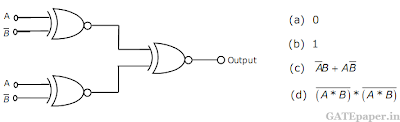Solution: https://www.youtube.com/watch?v=f86allXIvS0

2. The minimum number of NAND gates required to implement the Boolean function A + AB’ + AB’C is equal to
a. Zero
b. 1
c. 4
d. 7
Solution: https://www.youtube.com/watch?v=ZxI6mFEl4Hc

3. A switch-tail ring counter is made by using a single D flip flop. The resulting circuit is a
a. SR flip flop
b. JK flip flop
c. D flip flop
d. T flip flop
Solution: https://www.youtube.com/watch?v=NgHdSqy1YKw

4. When a CPU is interrupted, it
a. Stops executing of instructions
b. Acknowledges interrupt and branches of subroutine
c. Acknowledges interrupt and continues
d. Acknowledges interrupt and waits for the next instruction from the interrupt device
Answer:
Solution:

5. The minimum number of MOS transistors required to make a dynamic RAM cell is
a. 1
b. 2
c. 3
d. 4
Solution: https://www.youtube.com/watch?v=0zh1pqRqIPY

6. An SR latch is a
a. Combinational circuit
b. Synchronous sequential circuit
c. One bit memory element
d. One clock delay element
Solution: https://www.youtube.com/watch?v=C8x2OcVIKh8

7. A ‘DMA’ transfer implies
a. Direct transfer of data between memory and accumulator
b. Direct transfer of data between memory and I/O devices without use of µP
c. Transfer of data exclusively within µP registers
d. A fast transfer of data between µP and I/O devices
Solution:

8. An ‘Assembler’ of a microprocessor is used for
a. Assembly of processors in a production line
b. Creation of new program’s using different modules
c. Translation of a program form assembly language to machine language
d. Translation of a higher level language into English text
Solution:

9. For a TTL gate, match the followingSolution: https://www.youtube.com/watch?v=TSWQwxxgVO4

10. For an ADC, match the followingSolution: https://www.youtube.com/watch?v=QS5jEa8E8r4

11. A ROM is used to implement the Boolean functions given below.a. What is the minimum size of the ROM required?
b. Determine the data in each location of the ROM

Solution: https://www.youtube.com/watch?v=kqnpFRi_gYQ

12. A hypothetical CPU has a parallel address bus, a parallel data bus, a RD and WR active LOW signals. Two ROMs of size 4K words each and two RAMs of sizes 16K and 8K words, respectively, are to be connected to the CPU. The memories are to be so connected that they fill the address space of the CU as per the memory map shown in the figure. Assuming tat chip select signals are active LOW.a. What is the number of lines in the address bus of the CPU?
b. Determine the values of address X, Y, Z and W as decimal numbers.
c. Using a 2 to 4 decoder and some additional gates, draw a circuit for the decoding logic.

Solution: https://www.youtube.com/watch?v=7xJmGpkvMAo

13. A ‘code converter’ is to be designed to convert from the BCD (5421) code to normal BCD (8421) code. The input BCD combinations for each digit are given below. A block diagram of the converter is shown in figure.
a. Draw K- map for outputs W, X, Y and Z
b. Obtain minimized expression for the outputs W, X, Y and Z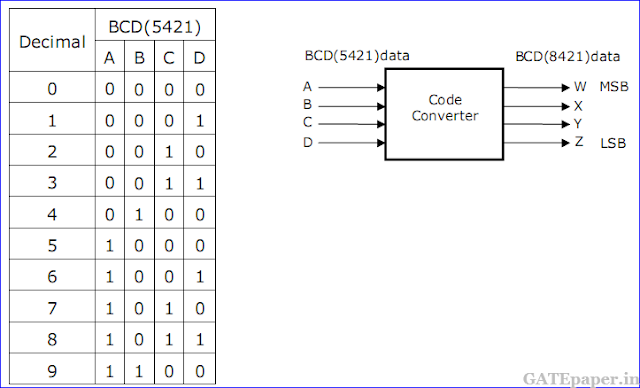Solution: https://www.youtube.com/watch?v=S-O75HI10qY

Name

Email *

Message *